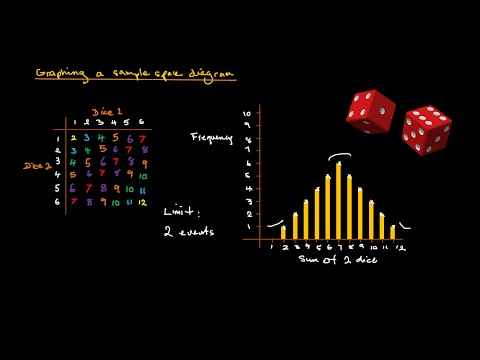Departments‎ > ‎

### 7th Grade Statistics and Probability Videos

Below are some videos that will support you in your learning and teaching the Statistics & Probability.  Also, I have attached the mini-lesson, guided lessons, examples, HW and Exit slips.  However, please. coordinate with Ms. T to discuss what is the best way that you want to present the material and break down the packets.  I am also here to support you should you have any questions.

Ms. Gomez

https://www.teachingchannel.org/videos/teaching-dependent-and-independent-events#Statistics - Dependent and Independent Events: 7th grade math This lesson teaches the distinction between Independent and Dependent Events, and how to calculate the probability of each.Probability explained | Independent and dependent events ... We give you an introduction to probability through the example of flipping a quarter and rolling a die. Practice this lesson yourself on KhanAcademy.org right now ...Probability - Sample Space Diagrams (independent events) Tutorial explaining what is meant by 'sample space' and how to draw and use a sample space diagram. Part 2 shows how to graph a sample space diagram as an in...Independent and Dependent Events in Probability Powered by http://www.tenmarks.com . Identify and find probability of independent and dependent events